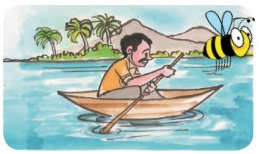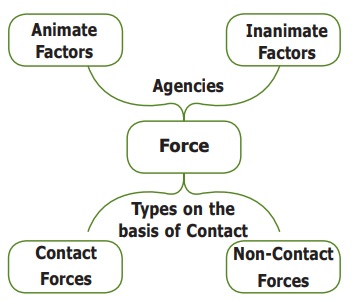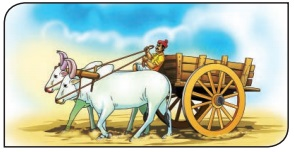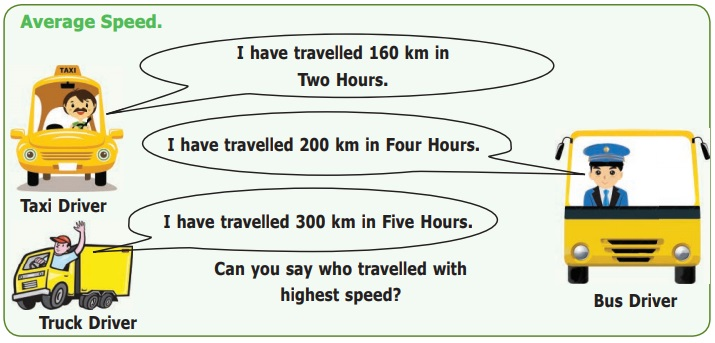Home | | Science 6th Std | Motion and Rest

# Motion and Rest

1. What is rest? What is motion? 2. Is Mohan in motion? 3. How things move? 4. What happens when we apply a force on an object? 5. Types of motion 6. Periodic and non-periodic motions 7. Speed Vs Slow ? 8. Uniform and non-uniform motion

Motion and Rest

1. What is rest? What is motion?

2. Is Mohan in motion?

3. How things move?

4. What happens when we apply a force on an object?

5. Types of motion

6. Periodic and non-periodic motions

7. Speed Vs  Slow ?

8. Uniform and non-uniform motion

1. What is rest? What is motion?

Suppose there is a book on your table right in the middle. Is the book moving? You will say “it is not moving; it is at rest”. If you push the book to one side of the table to clear space for keeping your notebook, then you will say the book is moving.

When the book was at the same place with respect to the table, you say the book was at rest; but when it was pushed from one place on the table to another place, you say it was moving.Activity 1

Can you identify whether it is push or pull that results in motion in the following cases?When there is a change of position of an object with respect to time, then it is called motion, if it remains stationary it is called rest.2. Is Mohan in motion?

Observe the following pictures and say whether Mohan is in motion or at restDiscuss: Who is correct? Is Mohan really in motion?We can readily observe that both Reka and Babu are correct. From the point of view of Babu, Mohan along with the bus is in motion; but for Reka who is sitting beside him, he is at one place; therefore stationary. So, according to Babu, Mohan is in motion; Mohan is at rest from Reka's observation. Can you think any other examples?Event 1: The man in the boat is moving with respect to the bank of river. He is at rest with respect to the boat.

Event 2:

The girl on the swing is rest with respect to the seat of the swing.

She is with moving respect to the garden.Event 3: Nisha is going to her grandmother's house by bicycle. The girl on the bicycle is moving with respect to the road.

She is rest with respect to the bicycle.Take the case of a book on a table at rest. Is it really without any motion? We know that Earth is rotating on its axis; therefore the table along with the book must be rotating. Is it not? We are also moving along with the earth. Therefore, from the point of view of ground on which we stand, the book is at ‘rest’. Similarly, while travelling in a speeding bus, we feel that the poles and trees seem to move backwards, and the things inside the bus are stationary.An object may appear to be stationary for one observer and appear to be moving for another. An object is at rest in relation to a certain set of objects and moving in relation to another set of objects. This implies that rest and motion are relative.

Activity 2

Moon or Cloud?

Observe the moon on a windy night with a fair bit of cloud cover in the sky. As a cloud passes in front of the moon you sometimes think it is the moon which is moving behind the cloud. What would you think if you were to observe a tree at the same time?It is a common illusion, caused by a combination of a bright moon and thin, dim clouds (usually moving fast). Your eye/brain has trouble processing difference of distance, especially in the sky, and so perceives the dim clouds as being further away than the bright moon.

Aryabatta, an ancient Indian astronomer, said that like the banks of the river appear to move back to a person in a boat floating gently in a river, the night sky studded with stars appear to move from east to west while Earth rotates from west to east. Learn more by asking others and reading up on your own.3. How things move?

When we kick a ball it moves. When we push the book on the table, it moves. When a bullock pulls the cart moves. Motion occurs when the object is pulled or pushed by an agency.In daily life, we pulled out water from the well, with bucket or “the animal pulls a bullock cart”. It is a person or animal, that is an animate agency that does the pushing or pulling.

Sometimes we see a tall grass in the meadow dancing in the wind, a piece of wood is moving down a stream. What pushes or pulls them? We know that blowing wind and flowing water is the cause. Sometimes the push or pull can be due to the inanimate agency.

Forces are push or pull by an animate or inanimate agency.

Contact, Non-Contact Forces

In all the above cases, the force is executed by touching the body. so, these type of force is called Contact Force.

Mysteriously ripen coconut falls to the ground. What pulls it to the ground? We would have heard of the ‘force of gravity’ of Earth. Gravity pulls the ripen coconut from the tree to the ground.Bring a magnet near the small iron nail. Suddenly the nail jumps into the air and sticks with the magnet. Observe that the magnet and the nail did not touch each other. Still, there was a pulling force that made the nail to jump towards the magnet. In these two examples, the force is applied without touching the object. Such forces are known as "non-contact forces"

Forces can be classified into two major types; contact and non-contact forces. Wind is making a flag flutter, a cart pulled by a bullock are contact forces. Magnetism, gravity are some examples of non-contact forces.4. What happens when we apply a force on an object?

What happens when you apply a force on an object? Say you push a book on the table. The book moves. Application of force in an object results in motion from a state of rest.What happens when a batsman hit a ball? The ball is already in motion, but with the strike, the speed of the ball increases. Moreover the direction of the ball changes. Application of force on object results in a change in its speed and change in its direction.

Crush a balloon, apply force on roti dough, pull a rubber band. In these cases the shape of the object change on application of force. Application of force in object results in expansion or contraction.Look at this picture. The person is applying force to stop the cart from moving. When the force is applied against the direction of the motion, the speed can be reduced, or even the motion stopped completely.Discuss what happens when you apply break in a speeding bicycle.

In a nutshell, the applied force is an interaction of one object on another that causes the second object to move from rest, or speed up, slow down, stop the motion, change the direction, compress or expand.

Forces can

1. Change the states of body from rest to motion or motion to rest.

2. Either change the speed or direction or both of the body.

3. Change the shape of the body.

Activity 35. Types of motion

Activity 4

Play with pencil

(i). Shanthi took a pencil and sharpened it with a sharpener. (ii) Then she drew a circle using the pencil and a compass. (iii) Later she took her ruler (scale) and drew a straight line in another paper. (iv) Then she kept the pencil between her finger and moved it back and forth.Now, look at the motion of the pencil in all these four cases. How was it?

i. In the first case, the pencil rotated in its axis.

ii. In the second case, it went in a circle.

iii. In the third case, the pencil travelled in a straight line.

iv. Fourth case, the pencil tip moved back and forth, that is it oscillated like a swing.

That is its motion was ‘rotational’ and then ‘circular’ ‘straight line or linear’ and later ‘oscillatory’.

Throw paper aeroplanes or paper dart. Watch its flight path when you throw it at an angle. The path curves i.e the paper flight is moving ahead but direction is changing while moving such paths are called curvilinear.6. Periodic and non-periodic motions

A fly buzzing around the room is a combination of all these and flight path is zigzag.You can classify the motion according to the path taken by the object.

a. Linear- moving in a straight line, like a person walking on a straight path, free fall.

b. Curvilinear - moving ahead but changing direction, like a throwing ball.

c. Circular -moving in a circle, swirling stone tied to the rope.

d. Rotatory -The movement of a body about its own axis, like a rotating top.

e. Oscillatory -coming back to the same position after a fixed time interval, like a pendulum.

f. Zigzag (irregular)- like the motion of a bee or people walking in a crowded street.

Oscillations at Greater Speed

Ask your friend to hold the two ends of a stretched rubber band. Strike it in the middle. Do you see it oscillates very very fast? When the oscillation is very swift, it is called as vibration.

Fast oscillations are referred to as vibrations.Activity 6

Classify the following according to the path it takes.

Linear ,Curvilinear, Circular,Rotatory,Oscillatory, Zigzag (irregular)

* A sprinter running a 100 m race

* A coconut falling from a tree

* striking a coin in a carom board game

* Motion of flies and mosquitoes

* Beating of heart

* Children playing in a swing

* The tip of hands of a clock

* Flapping of elephant’s ears

* A stone thrown into the air at an angle

* Movement of people in a bazaar

* Athlete running around a track

* Revolution of the moon around the earth

* The movement of a ball kicked in a football match

* Motion of a spinning top

* Revolution of the earth around the sun

* Swinging of a pendulum

* Children skidding on a sliding board

* Skidding down a playground slide

* Wagging tail of a dog

* Flapping of a flag in wind

* A car driving around a curve

* Woodcutter cutting with a saw

* Motion of water wave

* Motion of piston inside a syringe

* Bouncing ball

[add five motions you observe to this list

A sprinter running a 100 m race - Linear motion

A coconut falling from a tree - Linear-motion

Striking a coin in a carom board game - Linear motion

Motion of flies and mosquitoes - Zigzag (irregular) motion

Beating of heart - Oscillatory motion

Children playing in a swing - Oscillatory motion

The tip of hands of a clock - Circular motion

Flapping of elephant's ears - Oscillatory motion

A stone thrown into the air at an angle - Curvilinear motion

Movement of people in a bazaar - Zigzag (irregular) motion

Athlete running around a track - Circular motion

Revolution of the moon around the earth - Rotatory motion

The movement of a ball kicked in a football match - Curvilinear motion

Motion of a spinning top - Circular motion

Revolution of the earth around the sun - Rotatory motion

Swinging of a pendulum - Oscillatory motion

Children skidding on a sliding board - Linear motion

Skidding down a playground slide - Linear motion

Wagging tail of a dog - Oscillatory motion

Flapping of a flag in wind - Oscillatory motion

A car driving around a curve - Curvilinear motion

Woodcutter cutting with a saw - Linear motion

Motion of water wave - Linear motion

Motion of piston inside a syringe - Linear motion

Bouncing ball - Linear motion

Swirling stone tied to the rope - Circular motion

Person walking on a straight path - Linear motion

Throw paper aeroplanes at an angle - Curvilinear motion

Moving in a circle - Circular motion

Throwing ball - Curvilinear motion

7. Speed Vs  Slow ?

Take the case of the hour- hand of a clock. In one day it makes two rounds. Look at a bouncing ball. It bounces a certain number of times for a given time interval or period. Look at the water waves, in a given period that is a time interval; a fixed number of waves hit the shore.Motion repeated in equal intervals of time is called as periodic motion.

Let us take the example of sabing swing in wind. This motion is not in uniform interval. Such Motions are called non-periodic motion.

Can you notice an interesting fact? Do you notice that all oscillatory motions are periodic, but not all periodic are oscillatory?

Revolution of the moon around the earth is periodic but not oscillatory. However, the children playing in a swing is both periodic and oscillatory.

Look at the tall tree. When the wind is gentle, its branches are dancing slowly; but if the gentle wind becomes strong, the branches shake violently, and if the speed increases further, the branch may even break and fall. That is the motion can be slow or fast.

Can we say a motion is slow or fast without comparing anything?Compared to walking, cycling is fast, but a bus is faster than a cycle.

The aeroplane is much faster than a bus.

So, slow or fast is a relative concept which depends upon the motions we are comparing.

Then how to we say a body moves in a particular Speed?How do we say? Let us calculate how long they travelled in One Hour?

Distance travelled by the Car in One Hour = 80 Km (160/2)

Distance travelled by the Bus in One Hour = 50 Km (200/4)

Distance travelled by the Truck in One Hour = 60 Km (300/5)

Have you found out? say now.

Fastest Car, Slowest Bus.

Have you noticed that saying who is fast and slow? is easy when we calculate the distance they travelled in one hour.

Yes, it is easy when we calculate the distance they travelled in one hour.

The distance travelled by an object in unit time is called average speed of the object.

If an object travelled a distance (d) in time (t) then its

Average speed (s) is = distance travelled / time taken = d/t.

In other words, you divide the distance travelled by the time taken to get the speed.

Suppose a car travels 300 km in one hour. Then we say the speed of the car is ‘300 kmph’ (we read it as ‘three hundred kilometres per hour’).

If an object travelled 10 metre in 2 seconds, then

Average speed (s)

= distance travelled (d) / time taken (t)

= 10 metre/ 2 second

= 5 metre / second

bus takes three hours to cover this distance of 180 kilometres. Then its average speed is

Average speed (s)

= distance travelled (d) / time taken (t)

= 180 kilometre/ 3 hour

= 60 kilometre / hour

Please note that metre/second or kilometre/hour comes next to our answer for average speed. What is it?

Observe the formula for average speed. If we denote the distance in metre and time by seconds then the unit of average speed is metre/second.If we denote the distance in kilometre and time in hour then the unit of average speed is kilometre/hour.

Some times we use units like centimetre/second.

In science we generally use SI units. In SI units the unit of distance is metre and the unit of time is second. So, the SI unit of average speed is metre/second.

Let us  Calculate

1. A cat travelled 150 metres in 10 seconds, what is its average speed?

Solution:

Average speed (s) = distance travelled (d) / time taken (t)

= 150 metre/ 10 second

= 15 metre / second

2. Priya ride her bicycle 40 km in two hours. what is her average speed?

Solution:

Average speed (s) = distance travelled (d) / time taken (t)

= 40 km / 2 hour

= 20 km / hour

Our Speed...

Let us play a small game. Go to the playground with your friends. Mark 100 metre distance for a race. Conduct a friendly running race and calculate the time they taken to complete the distance by stopwatch. Now fill up the following table.Usain Bolt crossed 100metre in 9.58 seconds and made a world record. If you have the hope to run in a speed  above that speed. Then Olympic Gold Medal is waiting for you.If you know the speed and the time taken by the object travelled, then we can compute how much distance it had travelled?

Speed = distance travelled / time taken

(s = d/t)

s = d/t or st = d

therefore the distance travelled is speed × time.

If a ship travelled at a speed of 50 kmph and it sailed for five hours, how much distance it had travelled.

s = 50 kmph; t = 5 therefore

s × t = 50 kmph × 5 h = 250 km

If we know the speed and distance travelled we can compute the time taken.

s = d/t that is t = d/s

time taken = distance travelled / speed

Suppose a bus travels at a speed of 50 kmph and has to cover a distance of 300 km. How much time will it take?

t = d/s that is 300 km/50 kmph = 6 h.

Compute the following Numerical Problems.

1. If you travel 10 kilometres in 2 hours, your speed is 5 km km per hour.

2. If you travel 15 kilometres in 1/2 hour, you would travel 30 km km in one hour, and your speed is 30 km. km per hour.

3. If you run fast at 20 kilometres per hour for 2 hours, you will cover 40 Km

FACT FILE

A Cheetah is the fastest land animal running with an average speed of 112 km/h

8. Uniform and non-uniform motion

Suppose a train leaves Thiruchirapalli and arrives at Madurai. Is the train travelled in an uniform speed? First, the train was stationary. When the train left the station, the motion was slow and only after it left some distance that it gathered speed. After that it slowed down while crossing bridges and stop at intermediate stations for passengers. Finally, as the train approached Madurai, again, it slowed and finally came to a halt. That is the speed was not same all through the journey time. That is the speed was non-uniform. This motion is said to be non-uniform motion.

In a nutshell, we can classify the motion in terms a) path b) if it is periodic or not c) if the speed is uniform or not. However, in real life, the motions are combinations many types.However, in between the journey, there may have a stretch where in the train might have been going at a constant speed. During that interval the train was moving at uniform speed, that is uniform motion.

If an object covers uniform distances in uniform intervals then the motion of the object is called Uniform Motion. Otherwise the Motion is called Non-Uniform Motion.

Many motions we see in our day to day life are non-uniform. We will learn more about uniform and non-uniform motion in later classes.

Multiple Motion

Look at the bicycle. What type of motion does the wheel perform? What type of motion does the cycle in total perform?The tyres rotate and make a rotatory motion, but the cycle as such moves forward in a linear path.

Multiple Motion in a Sewing Machine* Motion of the needle linear motion

* Motion of the wheel circular motion

* Motion of footrest oscillatory motion

Tags : Forces and Motion | Term 1 Unit 2 | 6th Science , 6th Science : Term 1 Unit 2 : Forces and Motion
Study Material, Lecturing Notes, Assignment, Reference, Wiki description explanation, brief detail
6th Science : Term 1 Unit 2 : Forces and Motion : Motion and Rest | Forces and Motion | Term 1 Unit 2 | 6th Science